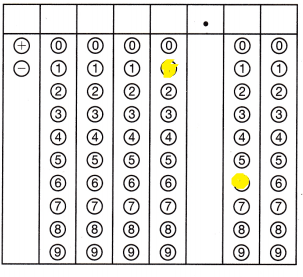Refer to our Texas Go Math Grade 8 Answer Key Pdf to score good marks in the exams. Test yourself by practicing the problems from Texas Go Math Grade 8 Module 12 Quiz Answer Key.

12.1-12.3 Properties of Translations, Reflections, and Rotations

Use the graph for Exercises 1-2.Question 1.
Graph the image of triangle ABC after a translation of 6 units to the right and 4 units down. Label the vertices of the image A’, B’, and C’.
The transformation is translation 6 units right and 4 unit down.
Apply the algebraic rule on the coordinates of vertices A, B and C to find vertices A’, B’ and C’.
(x, y) → (x+6, y – 4)
A(-4, 5) → A'(2, 1)
B(-4, 3) → B'(2, -1)
C(-1, 3) → C'(5, -1)Question 2.
On the same coordinate grid, graph the image of triangle ABC after a reflection across the y-axis. Label the vertices of the image A”, B”, and C”.
The transformation is reflection across the x-axis
Apply the algebraic rule on the coordinates of vertices A, B and C to find vertices A”, B” and C”.
(x, y) → (x, y)
A(-4, 5) → A”(-4, -5)
B(-4, 3) → B”(-4, -3)
C(-1, 3) → C”(-1, -3)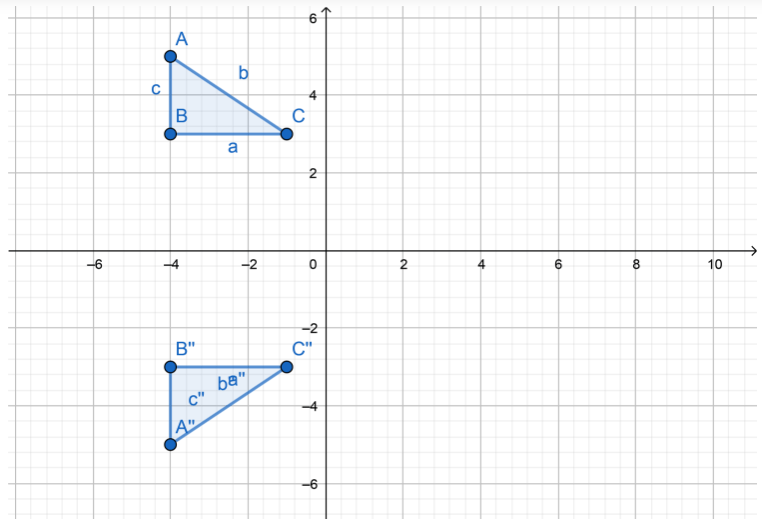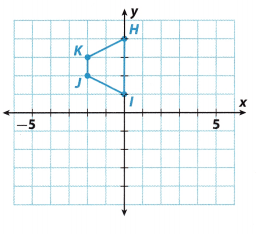Question 3.
Graph the image of trapezoid HIJK after it is rotated 180° about the origin. Label the vertices of the image. Find the vertices if the trapezoid HIJK is rotated 360°.
The transformation is rotation 180° about the origin. Apply the algebraic rule to find points H’, I’. J’ and K’.
The rute is (x, y) → (- x, y)
H(0, 4) → H'(0, -4)
I(0, 1) → I'(0, -1)
J(-2, 2) → J'(2, -2)
K(-2, 3) → K'(2, -3)Question 4.
Vocabulary Translations, reflections, and rotations produce a figure that is ________________ to the original figure.
Translations, reflections, and rotations produce a figure that is congruent to the original figure.

12.4 Algebraic Representations of Transformations

Question 5.
A triangle has vertices at (2, 3), (-2, 2), and (-3, 5). What are the coordinates of the vertices of the image after the translation (x, y) → (x + 4, y – 3)? Describe the transformation.
After the translation 4 units right and 3 units down, the coordinates of the vertices of the image are:
(x, y) → (x + 4, y – 3)
E(2, 3) → E'(6, 0)
F(-2, 2) → F'(2, -1)
G(-3, 5) → G'(1, 2)Essential Question

Question 6.
How can you use transformations to solve real-world problems?
Transformations are the simple functions in maths that are used when shifting the graphs to the left or right, upward or downward. the new graph is different from the pattern but it has some characteristics that are the same.
Example:
Suppose a country is passing through a big economic problem. Let us assume that many people are leaving the country for new opportunities. There will be many people that must remain in the country. So, this is an opportunity for them to start a new business even when the crisis is terrible. If they succeed, when the crisis is over, the business will be prosperous. So they took advantage of a difficult problem transforming it into an opportunity.

Texas Go Math Grade 8 Module 12 Mixed Review Texas Test Prep Answer Key

Selected Response

Question 1.
What would be the orientation of the figure L after a translation of 8 units to the right and 3 units up?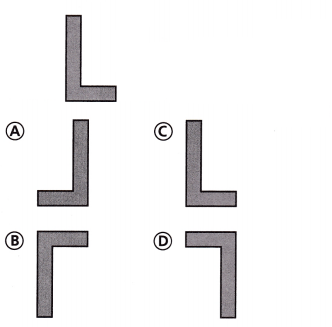(C)

Explanation:
After any translation, orientation of the image of the figure stays the same.
So, the solution is C, because that image has the same orientation like the original figure.

Question 2.
A straw has a diameter of 0.6 cm and a length of 19.5 cm. What is the surface area of the straw to the nearest tenth? Use 3.14 for π.
(A) 5.5 cm2
(B) 11.7 cm2
(C) 36.7 cm2
(D) 37.3 cm2
Explanation:
Given,
Straw has a diameter of 0.6 cm and a length of 19.5 cm.
Diameter= 0.6 cm
Lenght = 19.5 cm
We know that,
Surface Area = 2πrh + 2πr²
Area = 2×3.14×0.3×19.5 + 2×3.14(0.3)²
Area = 36.738 + 0.5652
Area = 37.3 sq. cm
Thus the correct answer is option A.

Question 3.
In what quadrant would the triangle be located after a rotation of 270° clockwise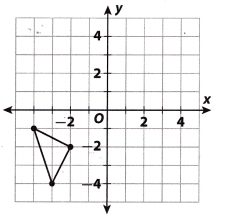(A) I
(B) II
(C) III
(D) IV
(D) IV

Explanation:
After a rotation of 90° counterclockwise about the origin, the triangle will be in IV quadrant. So, the solution is D.

Question 4.
Which rational number is greater than -3$$\frac{1}{3}$$ but less than –$$\frac{4}{5}$$?
(A) -0.4
(B) –$$\frac{9}{7}$$
(C) -0.19
(D) –$$\frac{22}{5}$$
(B) –$$\frac{9}{7}$$

Explanation:
Determine the least common multiple of the denominators:
LCM(3, 5, 7, 10, 100) = 2100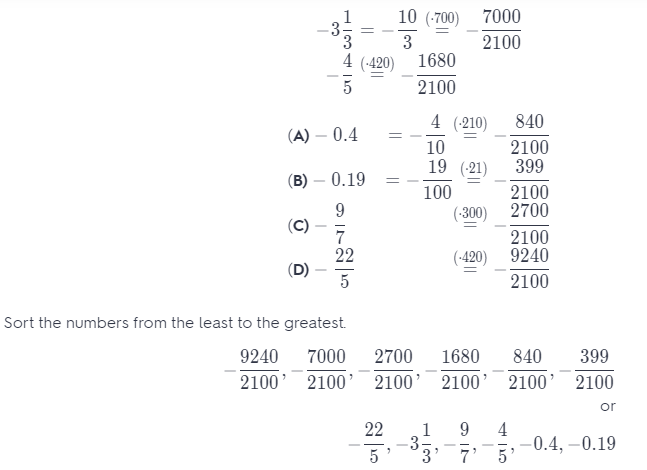Between -3$$\frac{1}{3}$$ and –$$\frac{4}{5}$$ is the number –$$\frac{9}{7}$$.
So, the solution is B.

Question 5.
Which of the following is not true of a trapezoid that has been reflected across the x-axis?
(A) The new trapezoid is the same size as the original trapezoid.
(B) The new trapezoid is the same shape as the original trapezoid.
(C) The new trapezoid is in the same orientation as the original trapezoid.
(D) The x-coordinates of the new trapezoid are the same as the x-coordinates of the original trapezoid.
(C) The new trapezoid is in the same orientation as the original trapezoid.

Explanation:
When you reflect a trapezoid across the x-axis, y-coordinates change and x-coordinates are the same as the x coordinates of the original trapezoid. The new trapezoid has the same size and shape as the original trapezoid.
So, the answer is C, because trapezoids don’t have the same orientation.

Question 6.
A triangle with coordinates (6, 4), (2, -1), and (-3, 5) is translated 4 units left and rotated 180° about the origin. What are the coordinates of its image?
(A) (2, 4), (-2, -1), (-7, 5)
(B) (4, 6), (-1, 2), (5, -3)
(C) (4, -2), (-1, 2), (5, 7)
(D) (-2, -4), (2, 1), (7, -5)
(D) (-2, -4), (2, 1), (7, -5)

Explanation:
Apply the rule (x, y) → (x – 4, y) for the translation 4 units left.
(6, 4) → (2, 4)
(2, -1) → (-2, -1)
(-3, 5) → (-7, 5)
Apply the rule (x, y) → (-x, -y) for the 180° rotation about the origin
(2, 4) → (-2, -4)
(-2, -1) → (2, 1)
(-7, 5) → (7, -5)
The coordinates of the image are (- 2, -4), (2, 1), (7, -5)
So, the correct answer is D.

Gridded Response

Question 7.
Solve the equation 3y + 17 = -2y + 25 for y.